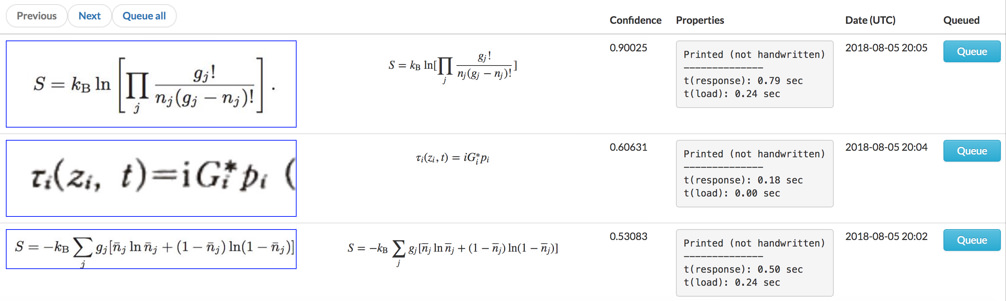# Extract text from images

Mathpix OCR is an API for
extracting text and math from images.

## Works especially well on small images

\left( \begin{array} c t ^ { \prime } \\ x ^ { \prime } \\ y ^ { \prime } \\ z ^ { \prime } \end{array} \right) = \left( \begin{array} { c c c c } { \gamma } & { - \gamma \beta } & { 0 } & { 0 } \\ { - \gamma \beta } & { \gamma } & { 0 } & { 0 } \\ { 0 } & { 0 } & { 1 } & { 0 } \\ { 0 } & { 0 } & { 0 } & { 1 } \end{array} \right) \left( \begin{array} c t \\ x \\ y \\ z \end{array} \right)

\Gamma _ { \epsilon } ( x ) = [ 1- e ^ { - 2\pi \epsilon } ] ^ { 1- x } \prod _ { n = 0} ^ { \infty } \frac { 1- \operatorname{exp} ( - 2\pi \epsilon ( n + 1) ) } { 1- \operatorname{exp} ( - 2\pi \epsilon ( x + n ) ) }

\ell ( \theta ) = \sum _ { i = 1} ^ { m } \operatorname{log} p ( x ; \theta )

6 \mathrm { CO } _ { 2 } + 6 \mathrm { H } _ { 2 } \mathrm { O } \rightarrow \mathrm { C } _ { 6 } \mathrm { H } _ { 12 } \mathrm { O } _ { 6 } + 6 \mathrm { O } _ { 2 }

We can also read large chunks of text that contain multiple lines. This is really helpful for when you want to extract a chunk of text from a PDF because you are unable to copy the source text directly.

Try us on your handwritten notes!

Powered by:

- machine learning

- deep learning

- computer vision

- big data

## Understand how your customers use OCR features in your app.

The mathpix API visualization dashboard gives you insights into how your customers are using your app.

You can also use the dashboard to tell us what images our recognition can get better at.• and more ...

## Ready to get started?

Choose the plan that’s right for you and your team.

• ### Monthly Pay As You Go

• First 1000 requests free
• $0.004/request (1-100K requests) •$0.002/request (100-300K requests)
• $0.001/request (300K+ requests) •$99/month Dashboard (optional)
• ### Enterprise

• Long term contracts
• Discounted prices for high volume
• 24/7 support
• Custom recognition features
• SLAs